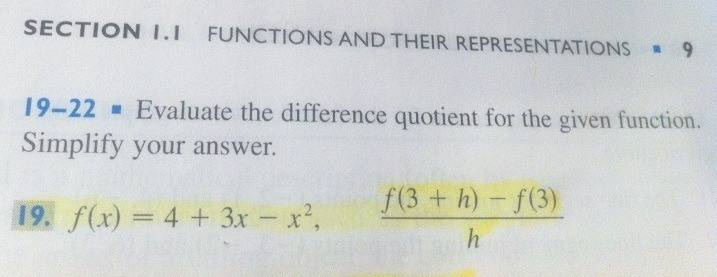# Simple difference quotient question. Function -> simplify answer

nukeman
Simple difference quotient question. Function --> simplify answer

## Homework Statement

I just don't understand this question! Here, I will take a picture of it. Can someone please explain how I answer these? Or even show me a website that shows how to do these? Its not in my review book.

QUESTION is in ATTACHMENTS!

## The Attempt at a Solution

#### Attachments

•photo (2).JPG
53.8 KB · Views: 627

Staff Emeritus
Homework Helper

It seems pretty straightforward what the question is asking. What specifically don't you understand?

nukeman

Can you tell me the first step? When you look at this, what is the first thing you see/do?

Staff Emeritus
Homework Helper

Exactly what the problem statement says: evaluate the difference quotient. If you don't understand what that means, try looking up "difference quotient" in your book.

nukeman

Must be missing something here.

I would plug in the f(x) values to the 2nd function, but there is no x's, just h ? How do I plug in values?

Mentor

The problem is asking you to evaluate the difference quotient to find f'(3).

nukeman

wow I'm lost. :(

Macch

Dude, f(x) = f(3+h) if x = 3+h

Mentor

wow I'm lost. :(
The difference quotient to find the derivative of a function, f'(x), looks like this:

$$f'(x) = \lim_{h \to 0} \frac{f(x + h) - f(x)}{h}$$

The difference quotient to find the derivative of a function at a specific number a, f'(a), looks like this:

$$f'(a) = \lim_{h \to 0} \frac{f(a + h) - f(a)}{h}$$

Staff Emeritus
Homework Helper
Gold Member

## Homework Statement

I just don't understand this question! Here, I will take a picture of it. Can someone please explain how I answer these? Or even show me a website that shows how to do these? Its not in my review book.
Here's the image:***************************

So, if $f(x)=4+3x-x^2\,,$ then what is $f(3+h)\ ?$

nukeman

Here's the image:***************************

So, if $f(x)=4+3x-x^2\,,$ then what is $f(3+h)\ ?$

SammyS, that is the one part I am having trouble fully understanding....

Staff Emeritus
Homework Helper

It would have helped if you could have said that right from the beginning.

In f(x)=4+3x-x2, the x is what's called a dummy variable. Anything you put in the lefthand side where the x is shows up wherever the x appears on the righthand side. For example, suppose you have g(x) = 2x+4. Then
\begin{align*}
g(y) = 2y+4 \\
g(h+3) = 2(h+3)+4 \\
g(4z) = 2(4z)+4 \\
g(weasel) = 2(weasel)+4 \\
g(3) = 2(3)+4 = 10
\end{align*}
Now tell us what you think f(3+h) is equal to in your problem.

Mentor

SammyS, that is the one part I am having trouble fully understanding....
Apparently you don't understand how to evaluate a function's formula.

If g(t) = 3 - t2 (for example)
then g(1) = 3 - (1)2 = 3 - 1 = 2
and g(5) = 3 - (5)2 = 3 - 25 = -23
and g(a + 2) = 3 - (a + 2)2

So for your function, what is f(3 + h)?

nukeman

"So for your function, what is f(3 + h)?"

f(3+h) = 4 + 3(3+h) - (3+h)^2 ?

Mentor

Yes. You'll need to expand the right side and collect common terms.

Staff Emeritus